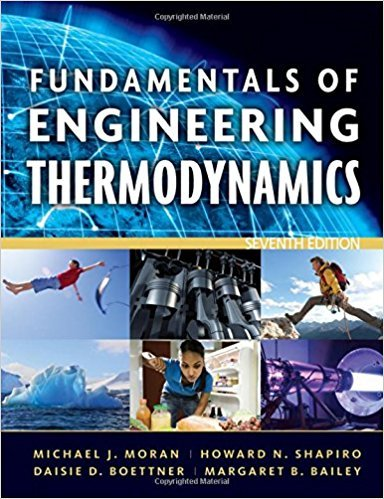×
Get Full Access to Fundamentals Of Engineering Thermodynamics - 7 Edition - Chapter 3 - Problem 98p
Get Full Access to Fundamentals Of Engineering Thermodynamics - 7 Edition - Chapter 3 - Problem 98p

×

# Using Generalized Compressibility DataFive lbmol of carbonISBN: 9780470495902 50

## Solution for problem 98P Chapter 3

Fundamentals of Engineering Thermodynamics | 7th Edition

• Textbook Solutions
• 2901 Step-by-step solutions solved by professors and subject experts
• Get 24/7 help from StudySoup virtual teaching assistantsFundamentals of Engineering Thermodynamics | 7th Edition

4 5 1 235 Reviews
14
0
Problem 98P

Problem 98P

Using Generalized Compressibility Data

Five lbmol of carbon dioxide (CO2), initially at 320 lbf/in.2, 660°R, is compressed at constant pressure in a piston-cylinder assembly. For the gas, W = −2000 Btu. Determine the final temperature, in °R.

Step-by-Step Solution:

Solution 98P

Step 1 of 3

For a 5 lb mol ofundergoes a constant pressure compression process in a piston cylinder assembly . The work , pressure and initial gas temperature are known , so here we need to find the final temperature.

Let us assume that theis a closed system and the pressure remains constant during the compression process.

Given,Step 2 of 3

Step 3 of 3

##### ISBN: 9780470495902

Unlock Textbook Solution